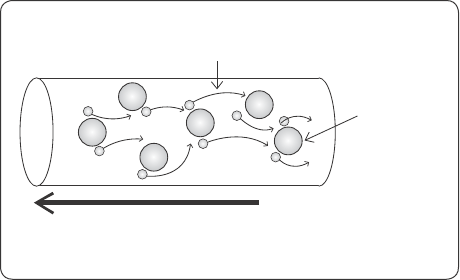The Triboelectric Series 51
The Triboelectric Series
Static electricity is more easily generated as the air gets drier—humidity prevents static elec-
tricity from gathering on a surface. Also, some clothes easily become charged, while others
do not, depending on the material they are made from. Since silk has good water absor-
bency and contains much more moisture than synthetic fibers, it can reduce the occurrence
of static electricity.
The polarities of the charges that are generated by friction differ according to the
materials that are rubbed together. These differences are represented by the triboelectric
series. For example, if hair and cotton are rubbed together, the hair will become positively
charged and the cotton will become negatively charged, but for cotton and vinyl, the cotton
will become positively charged and the vinyl will become negatively charged.
The farther apart the materials are in the triboelectric series, the more static electric-
ity is generated between them, and the closer together the objects are in the triboelectric
series, the less static electricity is generated. In other words, you can reduce the occurrence
of static electricity by wearing clothes that are made of materials that are close together in
the triboelectric series.
Movement of Charge and Direction of Current
Lightning is also a result of static electricity. Lightning occurs when the static electricity that
is produced by the friction between hail and ice particles in a cloud discharges between the
cloud and ground. In the case of lightning, air (which is an insulator through which electricity
has difficulty flowing) exists between the positive and negative charges, so a discharge does
not easily occur.
When a large amount of charge builds up and the potential difference between the
positive and negative charges is extremely large, the insulation of the air suddenly breaks
down and an electrical discharge occurs. Electrical discharge is the phenomenon in which
charge flows continuously. This continuous flow of electricity is called current.
Triboelectric Series
Negative Positive
s
k
i
n
P
V
C
P
o
l
y
e
t
h
y
l
e
n
e
P
o
l
y
e
s
t
e
r
C
o
p
p
e
r
R
u
b
b
e
r
W
o
o
d
S
t
e
e
l
C
o
t
t
o
n
P
a
p
e
r
S
i
l
k
W
o
o
l
N
y
l
o
n
H
a
i
r
G
l
a
s
s
The triboelectric series52 Chapter 1 What Is Electricity?
Electric current flows from positive to negative. Scientists have discovered that the
movement of electrons, however, is from negative to positive. Therefore, the direction in
which the electrons move is actually opposite to the direction in which current flows.
The amount of current is represented by the quantity of electricity passing through a
wire in a second.
For example, when a charge of 1C passes through a given point, the current I can be
obtained by dividing the charge (Q) in coulombs by the time (t) in seconds as follows.
I =
Q
t
=
1C
1s
= 1A
Also, the number of electrons flowing at 1A can be obtained by dividing 1C by the
quantity of charge in 1 electron, as follows:
1C
1.602 × 10
−19
C/electron
= 6.24 × 10
18
electrons
In other words, when a current of 1A is flowing, there are 6.24 × 10
18
electrons flowing
per second.
Direction of cuent
Electron
Coer wire
-
-
-
-
Direction of current and direction of electron movement
-
-
-
-
- - -
-
-
-
-
-
-
the size of the cuent is the
q
uantity of electricity paing
through a wire in one second.
Size of the currentMovement of Charge and Direction of Cuent 53
The speed at which the electrons move is very slow—less than 1 cm per second.
However, the speed at which electrical motion is transmitted to neighboring electrons is the
same as the speed of light: 300,000 km per second. Therefore, the current also flows at
300,000 km per second (the speed of light).
Although electricity itself cannot be seen with the naked eye, heat or light is often
produced when current flows. Therefore, we know that electricity exists by observing the
phenomena caused by current.
Electrons Moving at le than
1 cm per second
Cuent flowing at
300,000 km per second
Nucleus
-
-
-
-
-
-
-
-
-
Speed of electrons and speed of current

Get The Manga Guide to Electricity now with the O’Reilly learning platform.

O’Reilly members experience books, live events, courses curated by job role, and more from O’Reilly and nearly 200 top publishers.